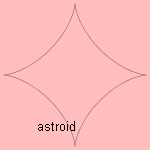# astroid

## rouletteThe astroid is the hypocycloid for which the rolled circle is four times as large as the rolling circle. The curve can be written in a Whewell equation as s = cos 2φ2). The curve can also be constructed as the envelope of the lines through the two points (cos t, 0) and (0, sin t), being a line piece between the axes of equal length. A mechanical device composed from a fixed bar with endings sliding on two perpendicular tracks is called a trammel of Archimedes. This is equivalent with a falling ladder, the astroid can also be seen as a glissette. The astroid is also the envelope of co-axial ellipses whoes sum of major and minor axes is constant. The length of the this unit astroid curve is 6, and its area is 3π/8. This sextic curve 3) is also called the regular star curve 4). 'Astroid' is an old word for 'asteroid', a celestial object in an orbit around the sun, intermediate in size between a meteoroid and a planet. The curve acquired its astroid name from a book from Littrow, published in 1836 in Vienna, replacing existing names as cubocycloid, paracycle and four-cusp-curve. Because of its four cusps it is also called the tetracuspid, and the hypocycloid of four cusps Abbreviation for a hypocycloid with four cusps (a=1/4) led to the name of H4. Some relations with other curves: its radial, pedal and orthoptic are all the same curve: the quadrifolium the curve is the catacaustic of the quadrifolium it is the deltoid's catacaustic it is the evolute of the ellipse The first to investigate the curve was Roemer (1674), during his search for gear teeth. Those who also worked on the curve where: Johann Bernoulli (1691) Leibniz (who corresponded in 1715) Johann Bernoulli (1725) d'Alembert (1748) The curve can be generalized into the super ellipse or Lamé curve. Some authors call this generalization the astroid. notes 1) In Italian: astroide. 2) φ: inclination angle of the tangent; s: arc length. 3) In Cartesian coordinates: (x2 + y2 - 1) + 27 x2 y2 = 0 4) Astrum (Lat.) = star